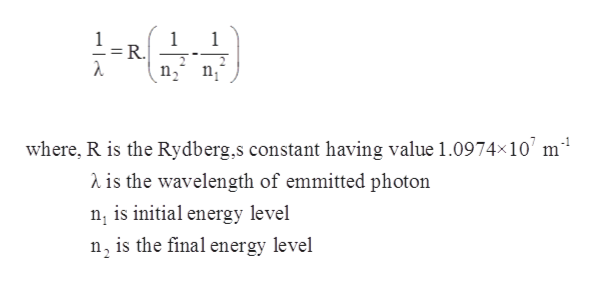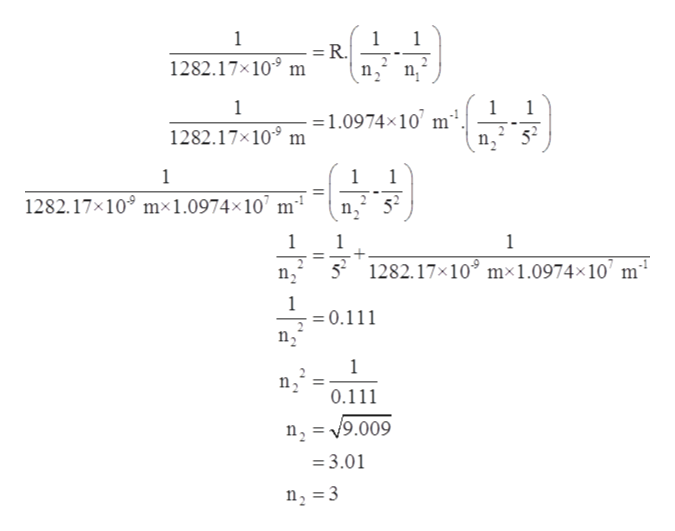# An electron in the n = 5 level of an H atom emits a photon of wavelength 1282.17 nm. To what energy level does the electron move? help

Question
7 views

An electron in the n = 5 level of an H atom emits a photon of wavelength 1282.17 nm. To what energy level does the electron move?

help

check_circle

Step 1

Given information:

Wavelength = 1282.17 nm

Initial state of an electron = 5

Step 2

Rydberg’s equation is used to calculate the wavelength of emitted photon which is given as follows:help_outlineImage Transcriptionclose1 = R. n2 n where, R is the Rydberg,s constant having value 1.0974x10 m1 is the wavelength of emmitted photon n is initial energy level n, is the final energy level fullscreen
Step 3

As, the light is emitted that means the transition of electron is taking place from high energy level to lower energy level thus, value of initial state is greater than fin...help_outlineImage Transcriptionclose1 1 = R 1282.17x109 m 2 1 1 = 1.0974x10' m. 1282.17x10 m 1 1 1282.17x109 mx1.0974x 10 m4 52 1 52 1282.17x109 mx1.0974x10 m4 1 =0.111 n2 1 n2 0.111 n2 =V9.009 =3.01 n2 3 fullscreen

### Want to see the full answer?

See Solution

#### Want to see this answer and more?

Solutions are written by subject experts who are available 24/7. Questions are typically answered within 1 hour.*

See Solution
*Response times may vary by subject and question.
Tagged in

### Chemistry